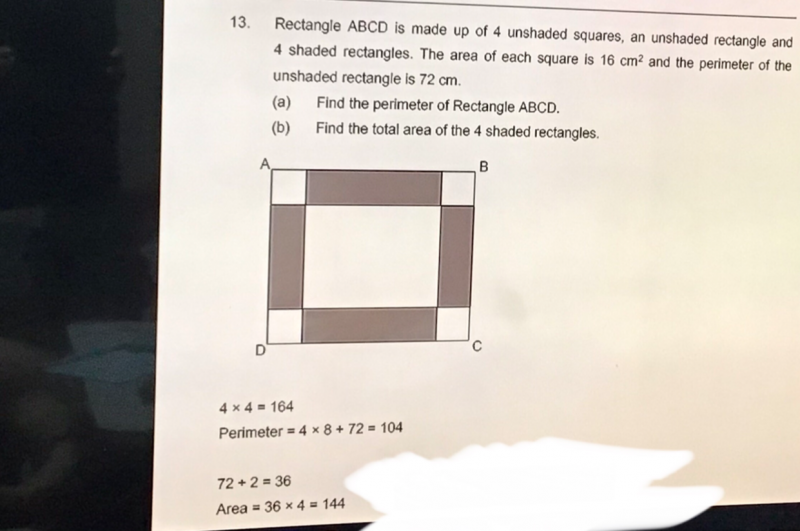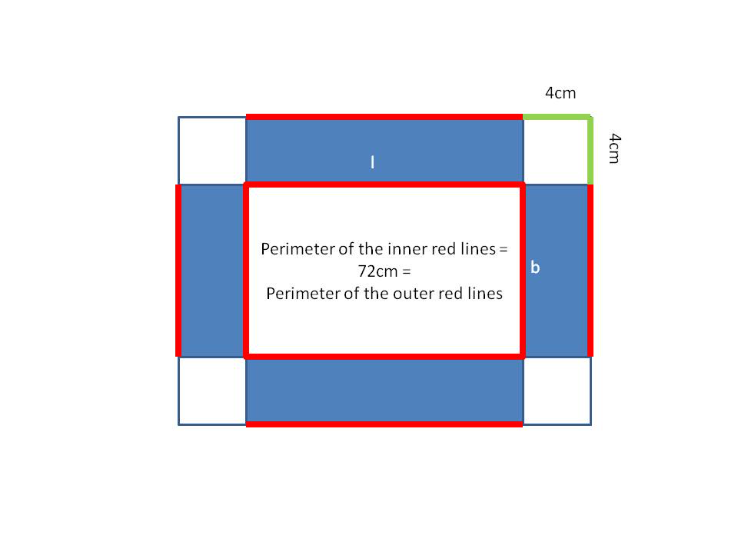# QuestionMay I ask why is the answer above use to solve this question?Alternative Solution:

(a)  Area of square = 16 => side = 4

Perimeter of 4  corners = 4 x (4+4) = 32

Perimeter of the rectangle ABCD = 72 + 32 = 104cm

(b) 2 (l + b) = 72 => l+b = 36

Area of 4 shaded rectangles = 2(l x 4) + 2 (b x 4) = 8l + 8b = 8(l + b ) = 8 x 36 =288 cm2

0 Replies 2 Likes ✔Accepted Answer

a. This answer is straight forward.

Side of small square = sqrt(16) = 4
This covers 8 sides of the perimeter, so 8 *4 = 32
The perimeter of the smaller rectangle is 72

So the perimeter of ABCD is 36 + 72 = 104 cm #

b. This is more tricky.  I think the given answer is wrong.

To get the total length of one shorter shaded bar and one longer shaded bar,
since the perimeter of the smaller rectangle is 72cm, it will be half of this perimeter.
So, 72/2 = 36
So the area of one shorter shaded bar and one longer shaded bar = 36 * 4 = 144
So the area of all 4 shaded bars = 144 * 2 = 288 cm2 #

Alternatively, you should just take the perimeter of the inner rectangle as also the length of of all 4 shaded rectangles.  Multiplied by the width of the small square, you get 72 * 4 = 288 cm2 #

0 Replies 1 Like